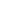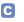bcParserObjC  1.1 Math Parser for Objective C, iOS and OSX
Class List
Here are the classes, structs, unions and interfaces with brief descriptions:Function defines the interface that describes a function that can be used as a user defined function in a MathParser instanceMathParser MathParser class provides the capability to parse and evaluate math expressions given as strings at runtimeInternal support protocol that represents parsed expression treeParameter protocol represents a parameter that is passed into a user defined functionParserException Exception thrown by the parser when the expression has a problemVariableDelegate returns the current value of a variable whose name is given